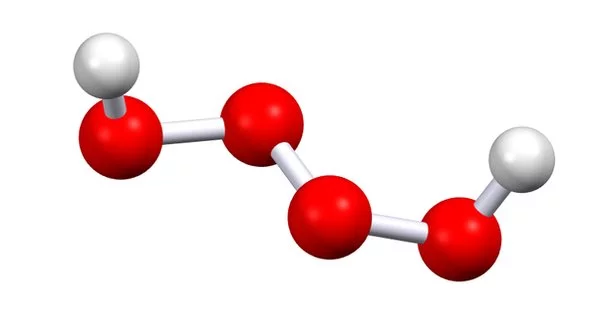Chemistry

# Pentaoxidane – an inorganic compoundPentaoxidane is an inorganic hydrogen and oxygen compound with the chemical formula H2O5. It is an oxide with five oxygen atoms in the molecule. This hydrogen polyoxide is one of the most unstable. The pentaoxidane molecule is made up of two hydrogen atoms and five oxygen atoms for a total of seven atoms.

Properties

• Chemical formula: H2O5
• Molar mass: 82.011 g·mol−1

Synthesis

• The compound is prepared as a byproduct of trioxidane production.
• H2O5 has also been synthesized by reaction among peroxy radicals at low temperature.

The molecular weight of pentaoxidane is determined by the sum of the atomic weights of each constituent element multiplied by the number of atoms, which is calculated to be:

82.01288 g/mol

The actual word for the above molecular weight is “molar mass,” which is dependent on each element’s atomic mass. The phrase “molecular weight” is an older name for “relative molar mass” or “molecular mass,” which is a dimensionless number equal to the molar mass divided by the molar mass constant defined as 1 g/mol. Molecular masses are determined using the normal atomic weights of each nuclide, whereas molar masses are calculated using the element’s atomic mass.